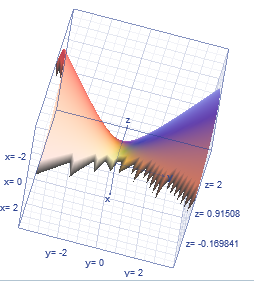# Draw a rough picture of the following surface and also clarify some basic geometric properties...

## Question:

Draw a rough picture of the following surface and also clarify some basic geometric properties (e.g. axis, vertex): {eq}x^2 + 2z^2 - y^2 = 1 {/eq}.

## Graph the function

We can observe the behavior of a function in the graph, if we have a function that depends on two variables {eq}(x,y) {/eq} the third variable is {eq}z {/eq} corresponding to the height.

We have, {eq}x^2 + 2z^2 - y^2 = 1 \\ 2z^2= y^{2}-x^{2}+1 \\ z=f(x,y)=\sqrt{ \frac{ y^{2}-x^{2}+1}{2} } \\ {/eq}

Graph the function {eq}f(x,y)=\sqrt{ \frac{ y^{2}-x^{2}+1}{2} } \\ {/eq}